# Mathematics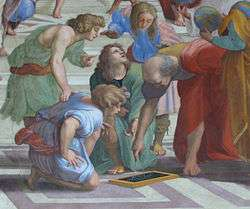Euclid (holding calipers), Greek mathematician, 3rd century BC, as imagined by Raphael in this detail from The School of Athens.

Mathematics (from Greek μάθημα máthēma, “knowledge, study, learning”) is the study of topics such as quantity (numbers), structure, space, and change. There is a range of views among mathematicians and philosophers as to the exact scope and definition of mathematics.

Mathematicians seek out patterns and use them to formulate new conjectures. Mathematicians resolve the truth or falsity of conjectures by mathematical proof. When mathematical structures are good models of real phenomena, then mathematical reasoning can provide insight or predictions about nature. Through the use of abstraction and logic, mathematics developed from counting, calculation, measurement, and the systematic study of the shapes and motions of physical objects. Practical mathematics has been a human activity from as far back as written records exist. The research required to solve mathematical problems can take years or even centuries of sustained inquiry.

Rigorous arguments first appeared in Greek mathematics, most notably in Euclid's Elements. Since the pioneering work of Giuseppe Peano (1858–1932), David Hilbert (1862–1943), and others on axiomatic systems in the late 19th century, it has become customary to view mathematical research as establishing truth by rigorous deduction from appropriately chosen axioms and definitions. Mathematics developed at a relatively slow pace until the Renaissance, when mathematical innovations interacting with new scientific discoveries led to a rapid increase in the rate of mathematical discovery that has continued to the present day.

Galileo Galilei (1564–1642) said, "The universe cannot be read until we have learned the language and become familiar with the characters in which it is written. It is written in mathematical language, and the letters are triangles, circles and other geometrical figures, without which means it is humanly impossible to comprehend a single word. Without these, one is wandering about in a dark labyrinth." Carl Friedrich Gauss (1777–1855) referred to mathematics as "the Queen of the Sciences". Benjamin Peirce (1809–1880) called mathematics "the science that draws necessary conclusions". David Hilbert said of mathematics: "We are not speaking here of arbitrariness in any sense. Mathematics is not like a game whose tasks are determined by arbitrarily stipulated rules. Rather, it is a conceptual system possessing internal necessity that can only be so and by no means otherwise." Albert Einstein (1879–1955) stated that "as far as the laws of mathematics refer to reality, they are not certain; and as far as they are certain, they do not refer to reality."

Mathematics is essential in many fields, including natural science, engineering, medicine, finance and the social sciences. Applied mathematics has led to entirely new mathematical disciplines, such as statistics and game theory. Mathematicians also engage in pure mathematics, or mathematics for its own sake, without having any application in mind. There is no clear line separating pure and applied mathematics, and practical applications for what began as pure mathematics are often discovered.

## History

The history of mathematics can be seen as an ever-increasing series of abstractions. The first abstraction, which is shared by many animals, was probably that of numbers: the realization that a collection of two apples and a collection of two oranges (for example) have something in common, namely quantity of their members.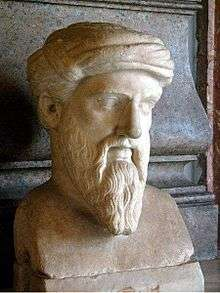Greek mathematician Pythagoras (c. 570 – c. 495 BC), commonly credited with discovering the Pythagorean theorem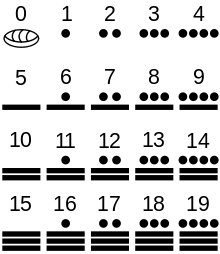As evidenced by tallies found on bone, in addition to recognizing how to count physical objects, prehistoric peoples may have also recognized how to count abstract quantities, like time – days, seasons, years.

Evidence for more complex mathematics does not appear until around 3000 BC, when the Babylonians and Egyptians began using arithmetic, algebra and geometry for taxation and other financial calculations, for building and construction, and for astronomy. The earliest uses of mathematics were in trading, land measurement, painting and weaving patterns and the recording of time.

In Babylonian mathematics elementary arithmetic (addition, subtraction, multiplication and division) first appears in the archaeological record. Numeracy pre-dated writing and numeral systems have been many and diverse, with the first known written numerals created by Egyptians in Middle Kingdom texts such as the Rhind Mathematical Papyrus.

Between 600 and 300 BC the Ancient Greeks began a systematic study of mathematics in its own right with Greek mathematics.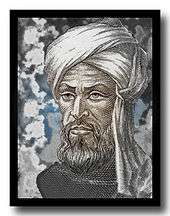Persian mathematician Al-Khwarizmi ( c. 780–c. 850 ), the inventor of the Algebra.

During the Golden Age of Islam, especially during the 9th and 10th centuries, mathematics saw many important innovations building on Greek mathematics: most of them include the contributions from Persian mathematicians such as Al-Khwarismi, Omar Khayyam and Sharaf al-Dīn al-Ṭūsī.

Mathematics has since been greatly extended, and there has been a fruitful interaction between mathematics and science, to the benefit of both. Mathematical discoveries continue to be made today. According to Mikhail B. Sevryuk, in the January 2006 issue of the Bulletin of the American Mathematical Society, "The number of papers and books included in the Mathematical Reviews database since 1940 (the first year of operation of MR) is now more than 1.9 million, and more than 75 thousand items are added to the database each year. The overwhelming majority of works in this ocean contain new mathematical theorems and their proofs."

### Etymology

The word mathematics comes from the Greek μάθημα (máthēma), which, in the ancient Greek language, means "that which is learnt", "what one gets to know", hence also "study" and "science", and in modern Greek just "lesson". The word máthēma is derived from μανθάνω (manthano), while the modern Greek equivalent is μαθαίνω (mathaino), both of which mean "to learn". In Greece, the word for "mathematics" came to have the narrower and more technical meaning "mathematical study" even in Classical times. Its adjective is μαθηματικός (mathēmatikós), meaning "related to learning" or "studious", which likewise further came to mean "mathematical". In particular, μαθηματικὴ τέχνη (mathēmatikḗ tékhnē), Latin: ars mathematica, meant "the mathematical art".

Similarly, one of the two main schools of thought in Pythagoreanism was known as the mathēmatikoi (μαθηματικοί) - which at the time meant "teachers" rather than "mathematicians" in the modern sense.

In Latin, and in English until around 1700, the term mathematics more commonly meant "astrology" (or sometimes "astronomy") rather than "mathematics"; the meaning gradually changed to its present one from about 1500 to 1800. This has resulted in several mistranslations: a particularly notorious one is Saint Augustine's warning that Christians should beware of mathematici meaning astrologers, which is sometimes mistranslated as a condemnation of mathematicians.

The apparent plural form in English, like the French plural form les mathématiques (and the less commonly used singular derivative la mathématique), goes back to the Latin neuter plural mathematica (Cicero), based on the Greek plural τα μαθηματικά (ta mathēmatiká), used by Aristotle (384–322 BC), and meaning roughly "all things mathematical"; although it is plausible that English borrowed only the adjective mathematic(al) and formed the noun mathematics anew, after the pattern of physics and metaphysics, which were inherited from the Greek. In English, the noun mathematics takes singular verb forms. It is often shortened to maths or, in English-speaking North America, math.

## Definitions of mathematics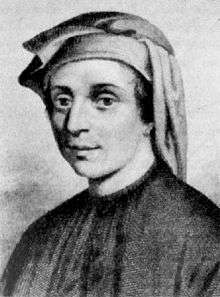Leonardo Fibonacci, the Italian mathematician who established the Hindu–Arabic numeral system to the Western World

Aristotle defined mathematics as "the science of quantity", and this definition prevailed until the 18th century. Starting in the 19th century, when the study of mathematics increased in rigor and began to address abstract topics such as group theory and projective geometry, which have no clear-cut relation to quantity and measurement, mathematicians and philosophers began to propose a variety of new definitions. Some of these definitions emphasize the deductive character of much of mathematics, some emphasize its abstractness, some emphasize certain topics within mathematics. Today, no consensus on the definition of mathematics prevails, even among professionals. There is not even consensus on whether mathematics is an art or a science. A great many professional mathematicians take no interest in a definition of mathematics, or consider it undefinable. Some just say, "Mathematics is what mathematicians do."

Three leading types of definition of mathematics are called logicist, intuitionist, and formalist, each reflecting a different philosophical school of thought. All have severe problems, none has widespread acceptance, and no reconciliation seems possible.

An early definition of mathematics in terms of logic was Benjamin Peirce's "the science that draws necessary conclusions" (1870). In the Principia Mathematica, Bertrand Russell and Alfred North Whitehead advanced the philosophical program known as logicism, and attempted to prove that all mathematical concepts, statements, and principles can be defined and proved entirely in terms of symbolic logic. A logicist definition of mathematics is Russell's "All Mathematics is Symbolic Logic" (1903).

Intuitionist definitions, developing from the philosophy of mathematician L.E.J. Brouwer, identify mathematics with certain mental phenomena. An example of an intuitionist definition is "Mathematics is the mental activity which consists in carrying out constructs one after the other." A peculiarity of intuitionism is that it rejects some mathematical ideas considered valid according to other definitions. In particular, while other philosophies of mathematics allow objects that can be proved to exist even though they cannot be constructed, intuitionism allows only mathematical objects that one can actually construct.

Formalist definitions identify mathematics with its symbols and the rules for operating on them. Haskell Curry defined mathematics simply as "the science of formal systems". A formal system is a set of symbols, or tokens, and some rules telling how the tokens may be combined into formulas. In formal systems, the word axiom has a special meaning, different from the ordinary meaning of "a self-evident truth". In formal systems, an axiom is a combination of tokens that is included in a given formal system without needing to be derived using the rules of the system.

### Mathematics as science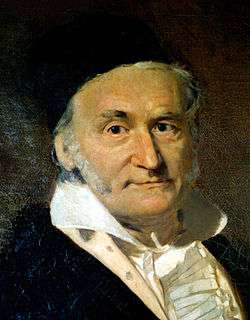Carl Friedrich Gauss, known as the prince of mathematicians

Gauss referred to mathematics as "the Queen of the Sciences". In the original Latin Regina Scientiarum, as well as in German Königin der Wissenschaften, the word corresponding to science means a "field of knowledge", and this was the original meaning of "science" in English, also; mathematics is in this sense a field of knowledge. The specialization restricting the meaning of "science" to natural science follows the rise of Baconian science, which contrasted "natural science" to scholasticism, the Aristotelean method of inquiring from first principles. The role of empirical experimentation and observation is negligible in mathematics, compared to natural sciences such as biology, chemistry, or physics. Albert Einstein stated that "as far as the laws of mathematics refer to reality, they are not certain; and as far as they are certain, they do not refer to reality." More recently, Marcus du Sautoy has called mathematics "the Queen of Science ... the main driving force behind scientific discovery".

Many philosophers believe that mathematics is not experimentally falsifiable, and thus not a science according to the definition of Karl Popper. However, in the 1930s Gödel's incompleteness theorems convinced many mathematicians that mathematics cannot be reduced to logic alone, and Karl Popper concluded that "most mathematical theories are, like those of physics and biology, hypothetico-deductive: pure mathematics therefore turns out to be much closer to the natural sciences whose hypotheses are conjectures, than it seemed even recently." Other thinkers, notably Imre Lakatos, have applied a version of falsificationism to mathematics itself.

An alternative view is that certain scientific fields (such as theoretical physics) are mathematics with axioms that are intended to correspond to reality. The theoretical physicist J.M. Ziman proposed that science is public knowledge, and thus includes mathematics. Mathematics shares much in common with many fields in the physical sciences, notably the exploration of the logical consequences of assumptions. Intuition and experimentation also play a role in the formulation of conjectures in both mathematics and the (other) sciences. Experimental mathematics continues to grow in importance within mathematics, and computation and simulation are playing an increasing role in both the sciences and mathematics.

The opinions of mathematicians on this matter are varied. Many mathematicians feel that to call their area a science is to downplay the importance of its aesthetic side, and its history in the traditional seven liberal arts; others feel that to ignore its connection to the sciences is to turn a blind eye to the fact that the interface between mathematics and its applications in science and engineering has driven much development in mathematics. One way this difference of viewpoint plays out is in the philosophical debate as to whether mathematics is created (as in art) or discovered (as in science). It is common to see universities divided into sections that include a division of Science and Mathematics, indicating that the fields are seen as being allied but that they do not coincide. In practice, mathematicians are typically grouped with scientists at the gross level but separated at finer levels. This is one of many issues considered in the philosophy of mathematics.

## Inspiration, pure and applied mathematics, and aesthetics

Main article: Mathematical beauty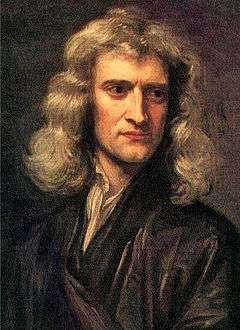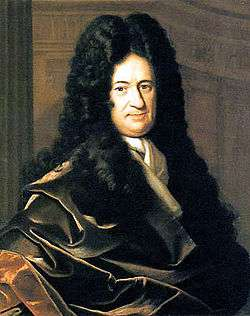Isaac Newton (left) and Gottfried Wilhelm Leibniz (right), developers of infinitesimal calculus

Mathematics arises from many different kinds of problems. At first these were found in commerce, land measurement, architecture and later astronomy; today, all sciences suggest problems studied by mathematicians, and many problems arise within mathematics itself. For example, the physicist Richard Feynman invented the path integral formulation of quantum mechanics using a combination of mathematical reasoning and physical insight, and today's string theory, a still-developing scientific theory which attempts to unify the four fundamental forces of nature, continues to inspire new mathematics.

Some mathematics is relevant only in the area that inspired it, and is applied to solve further problems in that area. But often mathematics inspired by one area proves useful in many areas, and joins the general stock of mathematical concepts. A distinction is often made between pure mathematics and applied mathematics. However pure mathematics topics often turn out to have applications, e.g. number theory in cryptography. This remarkable fact, that even the "purest" mathematics often turns out to have practical applications, is what Eugene Wigner has called "the unreasonable effectiveness of mathematics". As in most areas of study, the explosion of knowledge in the scientific age has led to specialization: there are now hundreds of specialized areas in mathematics and the latest Mathematics Subject Classification runs to 46 pages. Several areas of applied mathematics have merged with related traditions outside of mathematics and become disciplines in their own right, including statistics, operations research, and computer science.

For those who are mathematically inclined, there is often a definite aesthetic aspect to much of mathematics. Many mathematicians talk about the elegance of mathematics, its intrinsic aesthetics and inner beauty. Simplicity and generality are valued. There is beauty in a simple and elegant proof, such as Euclid's proof that there are infinitely many prime numbers, and in an elegant numerical method that speeds calculation, such as the fast Fourier transform. G.H. Hardy in A Mathematician's Apology expressed the belief that these aesthetic considerations are, in themselves, sufficient to justify the study of pure mathematics. He identified criteria such as significance, unexpectedness, inevitability, and economy as factors that contribute to a mathematical aesthetic. Mathematicians often strive to find proofs that are particularly elegant, proofs from "The Book" of God according to Paul Erdős. The popularity of recreational mathematics is another sign of the pleasure many find in solving mathematical questions.

## Notation, language, and rigor

Main article: Mathematical notation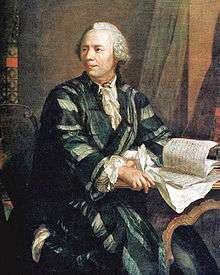Leonhard Euler, who created and popularized much of the mathematical notation used today

Most of the mathematical notation in use today was not invented until the 16th century. Before that, mathematics was written out in words, limiting mathematical discovery. Euler (1707–1783) was responsible for many of the notations in use today. Modern notation makes mathematics much easier for the professional, but beginners often find it daunting. It is compressed: a few symbols contain a great deal of information. Like musical notation, modern mathematical notation has a strict syntax and encodes information that would be difficult to write in any other way.

Mathematical language can be difficult to understand for beginners. Common words such as or and only have more precise meanings than in everyday speech. Moreover, words such as open and field have specialized mathematical meanings. Technical terms such as homeomorphism and integrable have precise meanings in mathematics. Additionally, shorthand phrases such as iff for "if and only if" belong to mathematical jargon. There is a reason for special notation and technical vocabulary: mathematics requires more precision than everyday speech. Mathematicians refer to this precision of language and logic as "rigor".

Mathematical proof is fundamentally a matter of rigor. Mathematicians want their theorems to follow from axioms by means of systematic reasoning. This is to avoid mistaken "theorems", based on fallible intuitions, of which many instances have occurred in the history of the subject. The level of rigor expected in mathematics has varied over time: the Greeks expected detailed arguments, but at the time of Isaac Newton the methods employed were less rigorous. Problems inherent in the definitions used by Newton would lead to a resurgence of careful analysis and formal proof in the 19th century. Misunderstanding the rigor is a cause for some of the common misconceptions of mathematics. Today, mathematicians continue to argue among themselves about computer-assisted proofs. Since large computations are hard to verify, such proofs may not be sufficiently rigorous.

Axioms in traditional thought were "self-evident truths", but that conception is problematic. At a formal level, an axiom is just a string of symbols, which has an intrinsic meaning only in the context of all derivable formulas of an axiomatic system. It was the goal of Hilbert's program to put all of mathematics on a firm axiomatic basis, but according to Gödel's incompleteness theorem every (sufficiently powerful) axiomatic system has undecidable formulas; and so a final axiomatization of mathematics is impossible. Nonetheless mathematics is often imagined to be (as far as its formal content) nothing but set theory in some axiomatization, in the sense that every mathematical statement or proof could be cast into formulas within set theory.

## Fields of mathematics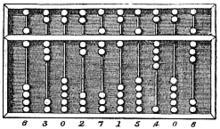An abacus, a simple calculating tool used since ancient times

Mathematics can, broadly speaking, be subdivided into the study of quantity, structure, space, and change (i.e. arithmetic, algebra, geometry, and analysis). In addition to these main concerns, there are also subdivisions dedicated to exploring links from the heart of mathematics to other fields: to logic, to set theory (foundations), to the empirical mathematics of the various sciences (applied mathematics), and more recently to the rigorous study of uncertainty. While some areas might seem unrelated, the Langlands program has found connections between areas previously thought unconnected, such as Galois groups, Riemann surfaces and number theory.

### Foundations and philosophy

In order to clarify the foundations of mathematics, the fields of mathematical logic and set theory were developed. Mathematical logic includes the mathematical study of logic and the applications of formal logic to other areas of mathematics; set theory is the branch of mathematics that studies sets or collections of objects. Category theory, which deals in an abstract way with mathematical structures and relationships between them, is still in development. The phrase "crisis of foundations" describes the search for a rigorous foundation for mathematics that took place from approximately 1900 to 1930. Some disagreement about the foundations of mathematics continues to the present day. The crisis of foundations was stimulated by a number of controversies at the time, including the controversy over Cantor's set theory and the Brouwer–Hilbert controversy.

Mathematical logic is concerned with setting mathematics within a rigorous axiomatic framework, and studying the implications of such a framework. As such, it is home to Gödel's incompleteness theorems which (informally) imply that any effective formal system that contains basic arithmetic, if sound (meaning that all theorems that can be proved are true), is necessarily incomplete (meaning that there are true theorems which cannot be proved in that system). Whatever finite collection of number-theoretical axioms is taken as a foundation, Gödel showed how to construct a formal statement that is a true number-theoretical fact, but which does not follow from those axioms. Therefore, no formal system is a complete axiomatization of full number theory. Modern logic is divided into recursion theory, model theory, and proof theory, and is closely linked to theoretical computer science, as well as to category theory. In the context of recursion theory, the impossibility of a full axiomatization of number theory can also be formally demonstrated as a consequence of the MRDP theorem.

Theoretical computer science includes computability theory, computational complexity theory, and information theory. Computability theory examines the limitations of various theoretical models of the computer, including the most well-known model – the Turing machine. Complexity theory is the study of tractability by computer; some problems, although theoretically solvable by computer, are so expensive in terms of time or space that solving them is likely to remain practically unfeasible, even with the rapid advancement of computer hardware. A famous problem is the "P = NP?" problem, one of the Millennium Prize Problems. Finally, information theory is concerned with the amount of data that can be stored on a given medium, and hence deals with concepts such as compression and entropy.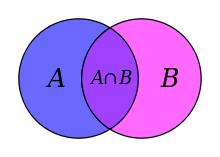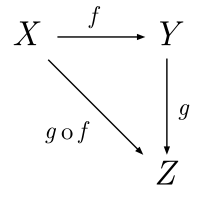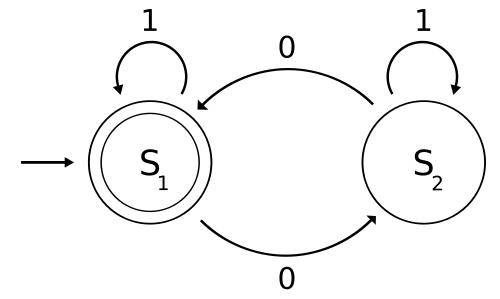Mathematical logic Set theory Category theory Theory of computation

### Pure mathematics

#### Quantity

The study of quantity starts with numbers, first the familiar natural numbers and integers ("whole numbers") and arithmetical operations on them, which are characterized in arithmetic. The deeper properties of integers are studied in number theory, from which come such popular results as Fermat's Last Theorem. The twin prime conjecture and Goldbach's conjecture are two unsolved problems in number theory.

As the number system is further developed, the integers are recognized as a subset of the rational numbers ("fractions"). These, in turn, are contained within the real numbers, which are used to represent continuous quantities. Real numbers are generalized to complex numbers. These are the first steps of a hierarchy of numbers that goes on to include quaternions and octonions. Consideration of the natural numbers also leads to the transfinite numbers, which formalize the concept of "infinity". According to the fundamental theorem of algebra all solutions of equations in one unknown with complex coefficients are complex numbers, regardless of degree. Another area of study is the size of sets, which is described with the cardinal numbers. These include the aleph numbers, which allow meaningful comparison of the size of infinitely large sets.Natural numbers Integers Rational numbers Real numbers Complex numbers

#### Structure

Many mathematical objects, such as sets of numbers and functions, exhibit internal structure as a consequence of operations or relations that are defined on the set. Mathematics then studies properties of those sets that can be expressed in terms of that structure; for instance number theory studies properties of the set of integers that can be expressed in terms of arithmetic operations. Moreover, it frequently happens that different such structured sets (or structures) exhibit similar properties, which makes it possible, by a further step of abstraction, to state axioms for a class of structures, and then study at once the whole class of structures satisfying these axioms. Thus one can study groups, rings, fields and other abstract systems; together such studies (for structures defined by algebraic operations) constitute the domain of abstract algebra.

By its great generality, abstract algebra can often be applied to seemingly unrelated problems; for instance a number of ancient problems concerning compass and straightedge constructions were finally solved using Galois theory, which involves field theory and group theory. Another example of an algebraic theory is linear algebra, which is the general study of vector spaces, whose elements called vectors have both quantity and direction, and can be used to model (relations between) points in space. This is one example of the phenomenon that the originally unrelated areas of geometry and algebra have very strong interactions in modern mathematics. Combinatorics studies ways of enumerating the number of objects that fit a given structure.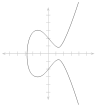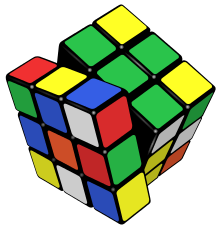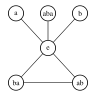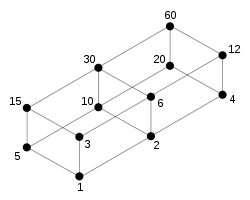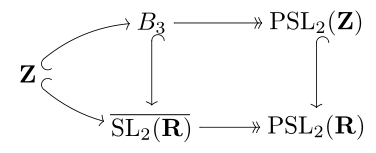Combinatorics Number theory Group theory Graph theory Order theory Algebra

#### Space

The study of space originates with geometry – in particular, Euclidean geometry, which combines space and numbers, and encompasses the well-known Pythagorean theorem. Trigonometry is the branch of mathematics that deals with relationships between the sides and the angles of triangles and with the trigonometric functions. The modern study of space generalizes these ideas to include higher-dimensional geometry, non-Euclidean geometries (which play a central role in general relativity) and topology. Quantity and space both play a role in analytic geometry, differential geometry, and algebraic geometry. Convex and discrete geometry were developed to solve problems in number theory and functional analysis but now are pursued with an eye on applications in optimization and computer science. Within differential geometry are the concepts of fiber bundles and calculus on manifolds, in particular, vector and tensor calculus. Within algebraic geometry is the description of geometric objects as solution sets of polynomial equations, combining the concepts of quantity and space, and also the study of topological groups, which combine structure and space. Lie groups are used to study space, structure, and change. Topology in all its many ramifications may have been the greatest growth area in 20th-century mathematics; it includes point-set topology, set-theoretic topology, algebraic topology and differential topology. In particular, instances of modern-day topology are metrizability theory, axiomatic set theory, homotopy theory, and Morse theory. Topology also includes the now solved Poincaré conjecture, and the still unsolved areas of the Hodge conjecture. Other results in geometry and topology, including the four color theorem and Kepler conjecture, have been proved only with the help of computers.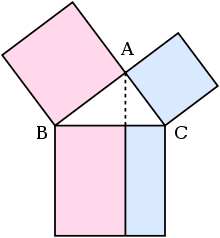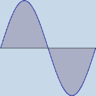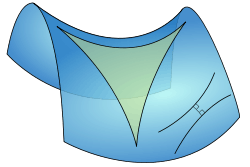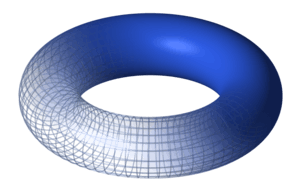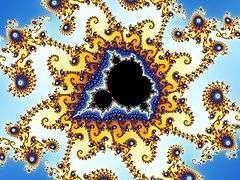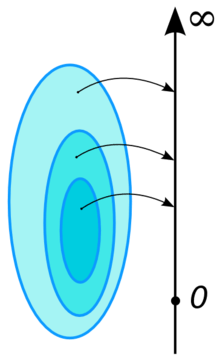Geometry Trigonometry Differential geometry Topology Fractal geometry Measure theory

#### Change

Understanding and describing change is a common theme in the natural sciences, and calculus was developed as a powerful tool to investigate it. Functions arise here, as a central concept describing a changing quantity. The rigorous study of real numbers and functions of a real variable is known as real analysis, with complex analysis the equivalent field for the complex numbers. Functional analysis focuses attention on (typically infinite-dimensional) spaces of functions. One of many applications of functional analysis is quantum mechanics. Many problems lead naturally to relationships between a quantity and its rate of change, and these are studied as differential equations. Many phenomena in nature can be described by dynamical systems; chaos theory makes precise the ways in which many of these systems exhibit unpredictable yet still deterministic behavior.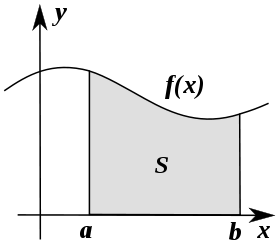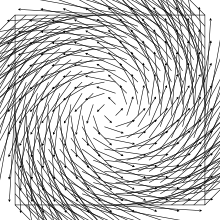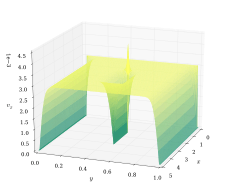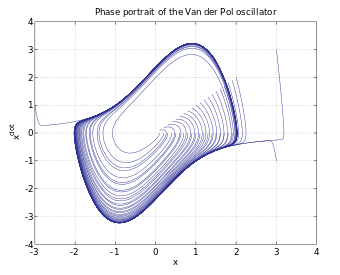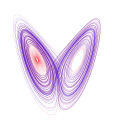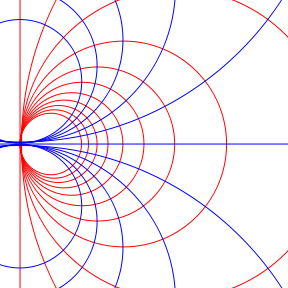Calculus Vector calculus Differential equations Dynamical systems Chaos theory Complex analysis

### Applied mathematics

Applied mathematics concerns itself with mathematical methods that are typically used in science, engineering, business, and industry. Thus, "applied mathematics" is a mathematical science with specialized knowledge. The term applied mathematics also describes the professional specialty in which mathematicians work on practical problems; as a profession focused on practical problems, applied mathematics focuses on the "formulation, study, and use of mathematical models" in science, engineering, and other areas of mathematical practice.

In the past, practical applications have motivated the development of mathematical theories, which then became the subject of study in pure mathematics, where mathematics is developed primarily for its own sake. Thus, the activity of applied mathematics is vitally connected with research in pure mathematics.

#### Statistics and other decision sciences

Applied mathematics has significant overlap with the discipline of statistics, whose theory is formulated mathematically, especially with probability theory. Statisticians (working as part of a research project) "create data that makes sense" with random sampling and with randomized experiments; the design of a statistical sample or experiment specifies the analysis of the data (before the data be available). When reconsidering data from experiments and samples or when analyzing data from observational studies, statisticians "make sense of the data" using the art of modelling and the theory of inference – with model selection and estimation; the estimated models and consequential predictions should be tested on new data.

Statistical theory studies decision problems such as minimizing the risk (expected loss) of a statistical action, such as using a procedure in, for example, parameter estimation, hypothesis testing, and selecting the best. In these traditional areas of mathematical statistics, a statistical-decision problem is formulated by minimizing an objective function, like expected loss or cost, under specific constraints: For example, designing a survey often involves minimizing the cost of estimating a population mean with a given level of confidence. Because of its use of optimization, the mathematical theory of statistics shares concerns with other decision sciences, such as operations research, control theory, and mathematical economics.

#### Computational mathematics

Computational mathematics proposes and studies methods for solving mathematical problems that are typically too large for human numerical capacity. Numerical analysis studies methods for problems in analysis using functional analysis and approximation theory; numerical analysis includes the study of approximation and discretization broadly with special concern for rounding errors. Numerical analysis and, more broadly, scientific computing also study non-analytic topics of mathematical science, especially algorithmic matrix and graph theory. Other areas of computational mathematics include computer algebra and symbolic computation.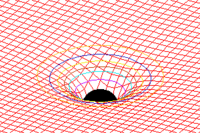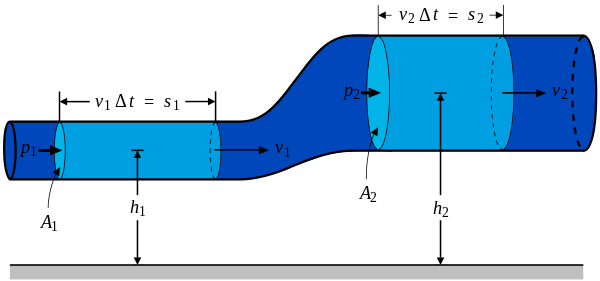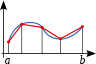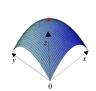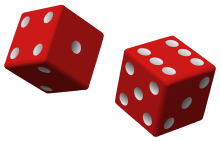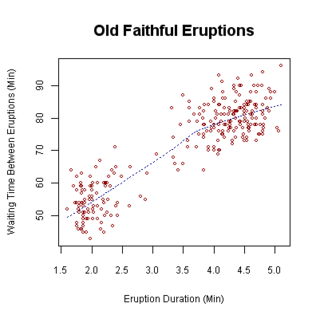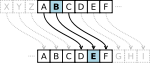Mathematical physics Fluid dynamics Numerical analysis Optimization Probability theory Statistics Cryptography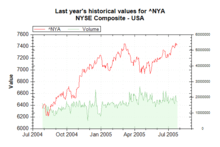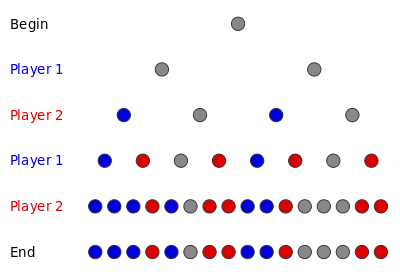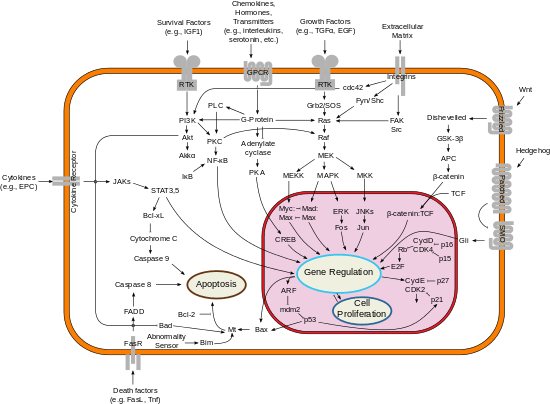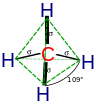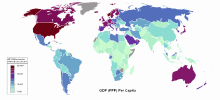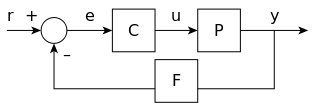Mathematical finance Game theory Mathematical biology Mathematical chemistry Mathematical economics Control theory

## Mathematical awards

Arguably the most prestigious award in mathematics is the Fields Medal, established in 1936 and awarded every four years (except around World War II) to as many as four individuals. The Fields Medal is often considered a mathematical equivalent to the Nobel Prize.

The Wolf Prize in Mathematics, instituted in 1978, recognizes lifetime achievement, and another major international award, the Abel Prize, was instituted in 2003. The Chern Medal was introduced in 2010 to recognize lifetime achievement. These accolades are awarded in recognition of a particular body of work, which may be innovational, or provide a solution to an outstanding problem in an established field.

A famous list of 23 open problems, called "Hilbert's problems", was compiled in 1900 by German mathematician David Hilbert. This list achieved great celebrity among mathematicians, and at least nine of the problems have now been solved. A new list of seven important problems, titled the "Millennium Prize Problems", was published in 2000. A solution to each of these problems carries a \$1 million reward, and only one (the Riemann hypothesis) is duplicated in Hilbert's problems.

1. No likeness or description of Euclid's physical appearance made during his lifetime survived antiquity. Therefore, Euclid's depiction in works of art depends on the artist's imagination (see Euclid).
2. "mathematics, n.". Oxford English Dictionary. Oxford University Press. 2012. Retrieved June 16, 2012. The science of space, number, quantity, and arrangement, whose methods involve logical reasoning and usually the use of symbolic notation, and which includes geometry, arithmetic, algebra, and analysis.
3. Kneebone, G.T. (1963). Mathematical Logic and the Foundations of Mathematics: An Introductory Survey. Dover. pp. 4. ISBN 0-486-41712-3. Mathematics ... is simply the study of abstract structures, or formal patterns of connectedness.
4. LaTorre, Donald R.; Kenelly, John W.; Biggers, Sherry S.; Carpenter, Laurel R.; Reed, Iris B.; Harris, Cynthia R. (2011). Calculus Concepts: An Informal Approach to the Mathematics of Change. Cengage Learning. p. 2. ISBN 1-4390-4957-2. Calculus is the study of change—how things change, and how quickly they change.
5. Ramana (2007). Applied Mathematics. Tata McGraw–Hill Education. p. 2.10. ISBN 0-07-066753-5. The mathematical study of change, motion, growth or decay is calculus.
6. Ziegler, Günter M. (2011). "What Is Mathematics?". An Invitation to Mathematics: From Competitions to Research. Springer. pp. 7. ISBN 3-642-19532-6.
7. Mura, Roberta (Dec 1993). "Images of Mathematics Held by University Teachers of Mathematical Sciences". Educational Studies in Mathematics. 25 (4): 375–385.
8. Tobies, Renate & Helmut Neunzert (2012). Iris Runge: A Life at the Crossroads of Mathematics, Science, and Industry. Springer. pp. 9. ISBN 3-0348-0229-3. It is first necessary to ask what is meant by mathematics in general. Illustrious scholars have debated this matter until they were blue in the face, and yet no consensus has been reached about whether mathematics is a natural science, a branch of the humanities, or an art form.
9. Steen, L.A. (April 29, 1988). The Science of Patterns Science, 240: 611–616. And summarized at Association for Supervision and Curriculum Development, www.ascd.org.
10. Devlin, Keith, Mathematics: The Science of Patterns: The Search for Order in Life, Mind and the Universe (Scientific American Paperback Library) 1996, ISBN 978-0-7167-5047-5
11. Eves
12. Marcus du Sautoy, A Brief History of Mathematics: 1. Newton and Leibniz, BBC Radio 4, September 27, 2010.
13. Waltershausen
14. Peirce, p. 97.
15. Hilbert, D. (1919–20), Natur und Mathematisches Erkennen: Vorlesungen, gehalten 1919–1920 in Göttingen. Nach der Ausarbeitung von Paul Bernays (Edited and with an English introduction by David E. Rowe), Basel, Birkhäuser (1992).
16. Einstein, p. 28. The quote is Einstein's answer to the question: "how can it be that mathematics, being after all a product of human thought which is independent of experience, is so admirably appropriate to the objects of reality?" He, too, is concerned with The Unreasonable Effectiveness of Mathematics in the Natural Sciences.
17. Peterson
18. Dehaene, Stanislas; Dehaene-Lambertz, Ghislaine; Cohen, Laurent (Aug 1998). "Abstract representations of numbers in the animal and human brain". Trends in Neuroscience. 21 (8): 355–361. doi:10.1016/S0166-2236(98)01263-6. PMID 9720604.
19. See, for example, Raymond L. Wilder, Evolution of Mathematical Concepts; an Elementary Study, passim
20. Kline 1990, Chapter 1.
21. "A History of Greek Mathematics: From Thales to Euclid". Thomas Little Heath (1981). ISBN 0-486-24073-8
22. Sevryuk 2006, pp. 101–109.
23. Both senses can be found in Plato. μαθηματική. Liddell, Henry George; Scott, Robert; A Greek–English Lexicon at the Perseus Project
24. Cipra, Barry A. (1982). "St. Augustine v. The Mathematicians". osu.edu. Ohio State University Mathematics department. Archived from the original on July 16, 2014. Retrieved July 14, 2014.
25. The Oxford Dictionary of English Etymology, Oxford English Dictionary, sub "mathematics", "mathematic", "mathematics"
26. "maths, n." and "math, n.3". Oxford English Dictionary, on-line version (2012).
27. James Franklin, "Aristotelian Realism" in Philosophy of Mathematics", ed. A.D. Irvine, p. 104. Elsevier (2009).
28. Cajori, Florian (1893). A History of Mathematics. American Mathematical Society (1991 reprint). pp. 285–6. ISBN 0-8218-2102-4.
29. Snapper, Ernst (September 1979). "The Three Crises in Mathematics: Logicism, Intuitionism, and Formalism". Mathematics Magazine. 52 (4): 207–16. doi:10.2307/2689412. JSTOR 2689412.
30. Peirce, Benjamin (1882). Linear Associative Algebra. p. 1.
31. Bertrand Russell, The Principles of Mathematics, p. 5. University Press, Cambridge (1903)
32. Curry, Haskell (1951). Outlines of a Formalist Philosophy of Mathematics. Elsevier. pp. 56. ISBN 0-444-53368-0.
33. Marcus du Sautoy, A Brief History of Mathematics: 10. Nicolas Bourbaki, BBC Radio 4, October 1, 2010.
34. Shasha, Dennis Elliot; Lazere, Cathy A. (1998). Out of Their Minds: The Lives and Discoveries of 15 Great Computer Scientists. Springer. p. 228.
35. Popper 1995, p. 56
36. Ziman
37. Johnson, Gerald W.; Lapidus, Michel L. (2002). The Feynman Integral and Feynman's Operational Calculus. Oxford University Press. ISBN 0-8218-2413-9.
38. Wigner, Eugene (1960). "The Unreasonable Effectiveness of Mathematics in the Natural Sciences". Communications on Pure and Applied Mathematics. 13 (1): 1–14. doi:10.1002/cpa.3160130102.
39. "Mathematics Subject Classification 2010" (PDF). Retrieved November 9, 2010.
40. Hardy, G.H. (1940). A Mathematician's Apology. Cambridge University Press. ISBN 0-521-42706-1.
41. Gold, Bonnie; Simons, Rogers A. (2008). Proof and Other Dilemmas: Mathematics and Philosophy. MAA.
42. Aigner, Martin; Ziegler, Günter M. (2001). Proofs from The Book. Springer. ISBN 3-540-40460-0.
43. "Earliest Uses of Various Mathematical Symbols". Retrieved September 14, 2014.
44. Kline, p. 140, on Diophantus; p. 261, on Vieta.
45. See false proof for simple examples of what can go wrong in a formal proof.
46. Ivars Peterson, The Mathematical Tourist, Freeman, 1988, ISBN 0-7167-1953-3. p. 4 "A few complain that the computer program can't be verified properly", (in reference to the Haken–Apple proof of the Four Color Theorem).
47. " The method of "postulating" what we want has many advantages; they are the same as the advantages of theft over honest toil." Bertrand Russell (1919), Introduction to Mathematical Philosophy, New York and London, p 71.
48. Patrick Suppes, Axiomatic Set Theory, Dover, 1972, ISBN 0-486-61630-4. p. 1, "Among the many branches of modern mathematics set theory occupies a unique place: with a few rare exceptions the entities which are studied and analyzed in mathematics may be regarded as certain particular sets or classes of objects."
49. Luke Howard Hodgkin & Luke Hodgkin, A History of Mathematics, Oxford University Press, 2005.
50. Clay Mathematics Institute, P=NP, claymath.org
51. Rao, C.R. (1997) Statistics and Truth: Putting Chance to Work, World Scientific. ISBN 981-02-3111-3
52. Like other mathematical sciences such as physics and computer science, statistics is an autonomous discipline rather than a branch of applied mathematics. Like research physicists and computer scientists, research statisticians are mathematical scientists. Many statisticians have a degree in mathematics, and some statisticians are also mathematicians.
53. Rao, C.R. (1981). "Foreword". In Arthanari, T.S.; Dodge, Yadolah. Mathematical programming in statistics. Wiley Series in Probability and Mathematical Statistics. New York: Wiley. pp. vii–viii. ISBN 0-471-08073-X. MR 607328.
54. Whittle (1994, pp. 10–11 and 14–18): Whittle, Peter (1994). "Almost home". In Kelly, F.P. Probability, statistics and optimisation: A Tribute to Peter Whittle (previously "A realised path: The Cambridge Statistical Laboratory upto 1993 (revised 2002)" ed.). Chichester: John Wiley. pp. 1–28. ISBN 0-471-94829-2.
55. Monastyrsky 2001: "The Fields Medal is now indisputably the best known and most influential award in mathematics."
56. Riehm 2002, pp. 778–782.
• Benson, Donald C., The Moment of Proof: Mathematical Epiphanies, Oxford University Press, USA; New Ed edition (December 14, 2000). ISBN 0-19-513919-4.
• Boyer, Carl B., A History of Mathematics, Wiley; 2nd edition, revised by Uta C. Merzbach, (March 6, 1991). ISBN 0-471-54397-7.—A concise history of mathematics from the Concept of Number to contemporary Mathematics.
• Davis, Philip J. and Hersh, Reuben, The Mathematical Experience. Mariner Books; Reprint edition (January 14, 1999). ISBN 0-395-92968-7.
• Gullberg, Jan, Mathematics – From the Birth of Numbers. W. W. Norton & Company; 1st edition (October 1997). ISBN 0-393-04002-X.
• Hazewinkel, Michiel (ed.), Encyclopaedia of Mathematics. Kluwer Academic Publishers 2000. – A translated and expanded version of a Soviet mathematics encyclopedia, in ten (expensive) volumes, the most complete and authoritative work available. Also in paperback and on CD-ROM, and online.
• Jourdain, Philip E. B., The Nature of Mathematics, in The World of Mathematics, James R. Newman, editor, Dover Publications, 2003, ISBN 0-486-43268-8.
• Maier, Annaliese, At the Threshold of Exact Science: Selected Writings of Annaliese Maier on Late Medieval Natural Philosophy, edited by Steven Sargent, Philadelphia: University of Pennsylvania Press, 1982.At Wikiversity, you can learn
more and teach others about Mathematics at the School of Mathematics.